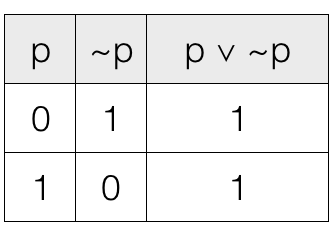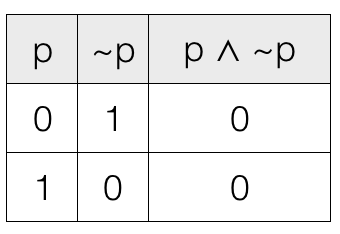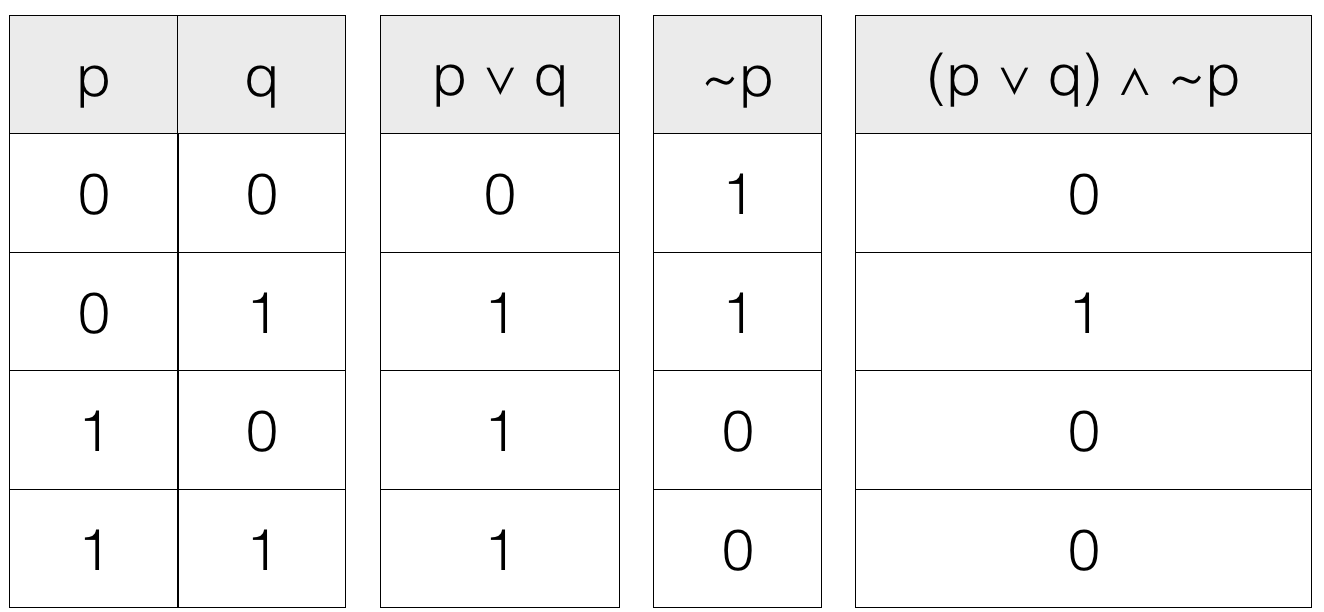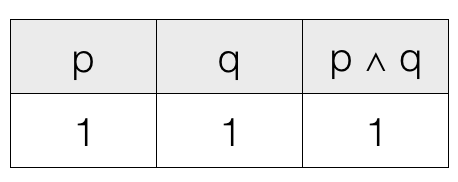# Propositional Logic Important Terms

Boolean Algebra

In this tutorial we will cover some important terms related to propositional logic.

## Tautology

A proposition (statement) is a tautology if it is logically true. That is, the propositions having nothing but 1s i.e., Ts in its truth table column. Where, 1 and T denotes True.

Example

The compound statement (proposition) consists of two simple propositions.
p = He is a YouTuber
and its negation
~p = He is not a YouTuber

And the two simple propositions are connected by an OR connective (Disjunctive operator). So, we can express the given compound proposition as
x = p ∨ ~p

That is, if any one of the simple proposition is true then the compound proposition is true.

Truth table of x = p ∨ ~pWe can see that the column contains only 1s i.e., True values. So, the given compound statement (proposition) is a tautology.

So, the statement "Either he is a Youtuber or he is not a Youtuber" is a tautology because the final result is true.

A proposition (statement) is a contradiction if it is logically false. That is, the propositions having nothing but 0s i.e., Fs in its truth table column. Where, 0 and F denotes False.

Example

The compound statement (proposition) consists of two simple propositions
p = He is a YouTuber
and its negation
~p = He is not a YouTuber

And the two simple propositions are connected by an AND connective (Conjunctive operator). So, we can express the given compound proposition as
x = p ∧ ~p

That is, if any one of the simple proposition is false then the compound proposition is false.

Truth table of x = p ∧ ~pWe can see that the column contains only 0s i.e., False values. So, the given compound statement (proposition) is a contradiction.

So, the statement "He is a Youtuber and he is not a Youtuber" is a contradiction because the final result is false.

## Contingent

A proposition is a contingent if it is logically true for some combination of truth values of its components and logically false for some other combination of truth values of its components.

That is, the propositions having some combination of 0s i.e., Fs and 1s i.e., Ts in its truth table column. Where 0 and F denotes False and 1 and T denotes True.

Example
He is either a YouTuber or a Developer, and he is not a YouTuber.

The compound statement (proposition) consists of three simple propositions
p = He is a YouTuber
q = He is a Developer
and negation of p
~p = He is not a YouTuber

Let us mark the statements.The first two simple propositions are connected by an OR connective (Disjunctive operator). So, we can express that part as x = p ∨ qAnd the third simple proposition ~p is connected by AND connective (Conjunctive Operator) with the compound proposition x. So, we can express that part as y = x ∧ ~p
y = (p ∨ q) ∧ ~pTruth table of y = (p ∨ q) ∧ ~pWe can see that the column contains 0s i.e., False values and 1s i.e., True values. So, the given compound statement (proposition) is a contingent.

## Consistent Statement

Two statements are said to be consistent if and only if their conjunction is not a contradiction.

Example
p = 10 greater than 5
q = March comes after January

So, we have two simple propositions (statement). We can call them consistent statements only if their conjunction (AND operation) is not a contradiction.

The first proposition p = true as 10 is greater than 5.

The second proposition q = true as March comes after January.1 means it is not a contradiction. So, p and q are consistent.

## Converse

The converse of a conditional proposition is another conditional proposition determined by interchanging the antecedent and consequent.

If we have a conditional proposition
p ⇒ q
then converse of the conditional proposition is
q ⇒ p

Example p = She is a programmer.
q = She writes code.

Then the conditional
p ⇒ q = If she is a programmer then she writes code.

And its converse will be a new conditional
q ⇒ p = If she writes code then she is a programmer.

## Inverse

The inverse of a conditional proposition is another conditional proposition having negated antecedent and consequent.

If we have a conditional proposition
p ⇒ q
then inverse of the conditional proposition is
~p ⇒ ~q

Example
p = He is a programmer.
q = He writes code.

And negation of the above statements
~p = He is not a programmer
~q = He does not write code

Then the conditional
p ⇒ q = If he is a programmer then he writes code.

And its inverse will be a new conditional
~p ⇒ ~q = If he is not a programmer then he does not write code.

## Contrapositive

The contrapositive of a conditional is another conditional whose antecedent is equal to the negated consequent of the first conditional and consequent is equal to the negated antecedent of the first conditional.

If we have a conditional proposition
p ⇒ q
then contrapositive of the conditional proposition is
~q ⇒ ~p

Example
p = It is night.
q = It is dark outside.

And negation of the above statements
~p = It is not night.
~q = It is not dark outside.

Then the conditional
p ⇒ q = If it is night then it is dark outside.

And its contrapositive will be a new conditional
~q ⇒ ~p = If it is not dark outside then it is not night.